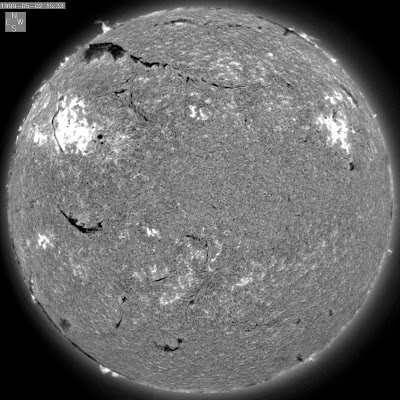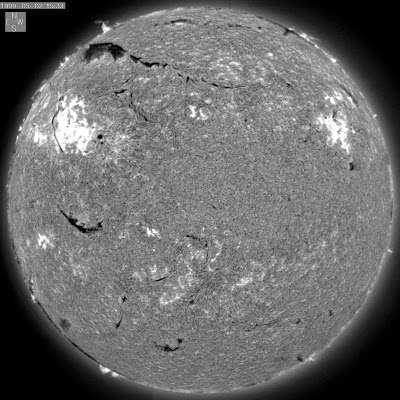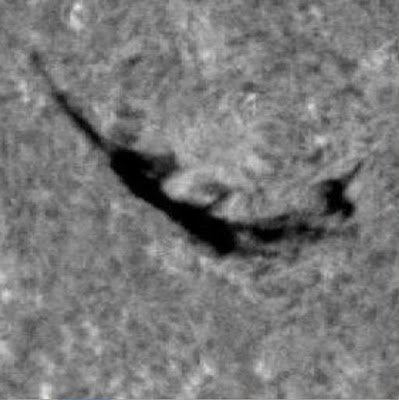## Saturday, 3 October 2015

### Testing the Compatibility of converted JPEGs with AAFDCC

Efforts continue to contact Dr. Bernasconi to request him to run his code on the converted images and see if they are compatible or have any issues.

### Complete Procedure 1.0

STEP1: RESIZE to 2032x2032

In the folder where you have all the original JPEG images:

for file in *.jpg; do convert \$file -resize 2032x2032 resized_\$file; done

STEP2: CONVERT to .fits files

a. Move the resized JPEGS to a new folder and convert them to .fits files:

for file in *.jpg; do convert \$file \$file.fits; done

b. Create a list of the resized_ filenames for the next step:

ls | grep -i "resized" > filenames.txt

STEP3:  Extract the TIME, DATE, Solar disk CENTER(X,Y) and disk RADIUS from resized JPEG images in MATLAB

<date_time_circles.m>

% 153= # of files
% 42= length of the string of each filename
% (including a return at the end of each filename)

yy=names(:,23:26);      % Year
mm=names(:,27:28);   % Month
dd=names(:,29:30);     % Day
hh=names(:,32:33);     % Hours
min=names(:,34:35);   % Minutes
ss=names(:,36:37);      % Seconds

for n=1:153
timeobs(n,:)=[hh(n,:),':',min(n,:),':',ss(n,:)];
dateobs(n,:)=[yy(n,:),'-',mm(n,:),'-',dd(n,:)];
date_obs(n,:)=[yy(n,:),'-',mm(n,:),'-',dd(n,:),'T',hh(n,:),':',min(n,:),':',ss(n,:),'.000Z'];
end

data=horzcat(timeobs,dateobs,date_obs);
dlmwrite('date_time.txt',data,'delimiter','');

% Extracting the Center of the Solar Disk and its radius:

for n=1:153
[centers(n,:), radii(n), metric(n)] = imfindcircles(imread(sprintf(names(n,1:end-1))), [950 1000], ...
'Sensitivity',0.9900,'EdgeThreshold',0.01,'Method','TwoStage','ObjectPolarity', 'Bright');
end

centers2=round(centers);
fileid=fopen('circle_info.txt','w')
for n=1:153
fprintf(fileid,'%d %d %d\n',centers2(n,1),centers2(n,2),centers2(n,3));
end
fclose(fileid);

This routine will take hours to run for more than 150 files.

Move the new files <date_time.txt> and <circle_info.txt> to the folder containing the converted .fits files

<date_time.txt>  should have a single column of stings joined together.

STEP4: Appending the header

a. Create a list of all file names for .fits files:

ls | grep -i "fits" > filenames.txt

b. Move the file <edit_header.py> to the directory containing all .fits files, <filenames.txt>, <date_time.txt> and <circle_info.txt>.

c. Change the number of filenames in the Python code to be equal to the entries you have in <filenames.txt>

d. Open a terminal and type:

And watch magic happen, the headers of all .fits files are changed!

## Thursday, 1 October 2015

### Known and Unknown parameters

Some parameter values in the header are the same for all images, and some of them don't matter for the analysis of the AAFDCC. So, these values will be added to the header of the converted fits files:

OBJECT='SUN'
TYPE-OBS='FULLDISK'
SEEING=0                  / I don't think this matters for the analysis
CDELT1=1.05440       / This quantity is the same for all images
CDELT2=1.05440       /
CTYPE1='ARCSEC'
CTYPE2='ARCSEC'
CRVAL1=0                / I don't really know what this means, but it is always 0
CRVAL2=0
ORIGIN='BBSO'
TELESCOPE='SINGER'
WAVELNTH='HALPHA'
OBSERVER='X'
EXPTIME=0.04000   / Observing times vary from 0.025 to 0.05 seconds, but it probably doesn't matter for AAFDCC. Even if it does, there is no way to derive the exposure time.
TEC_TEMP=0          / There is no way to extract this from the images
ASP=1.00000           / Raw image aspect ratio

The remaining parameters listed below can be extracted from the converted images:

# By analysis of the solar disk, detecting its center and radius:
CRPIX1=      / Central pixel in X
CRPIX2=      / Central pixel in Y
CENX=              / Raw image disk center X
CENY=              / Raw image disk center Y
WIDT=               / Width of the disk (pixels)
MAXC=              / Quiet Sun Max Brightness (probably won't be able to extract this)

# From the filename:
TIME-OBS='hh:mm:ss'  / From the filename
DATE-OBS='yyy-mm-dd'  / From filename
DATE_OBS='yyy-mm-ddThh:mm:ss.000Z' /

Now, the job is to actually extract these parameters and write them in the filename headers.

## Thursday, 25 June 2015

### JPEG to FITS

There are various ways to convert JPEG files to FITS files:

The easiest are:

1. Linux terminal:   convert  <filename.jpeg>   <filename.fits>

2. MATLAB:          import filename.jpeg;
fitswrite(filename,'new_filename.fits')

Problem:
Both these ways produce a default header with minimal information on the newly created fits file.

Example: A header from  .fts file from BBSO  (required):

SIMPLE  =                    T / Written by IDL:   6-Jul-2000 13:21:53.00
BITPIX  =                   16 /
NAXIS   =                    2 /
NAXIS1  =                 2032 /
NAXIS2  =                 2032 /
BZERO   =              0.00000 /
BSCALE  =              1.00000 /
OBJECT  = 'SUN'                /
TYPE-OBS= 'FULLDISK'           /
SEEING  =                    0 / VERY POOR
CRPIX1  =           1016.50000 /
CRPIX2  =           1016.50000 /
CDELT1  =              1.05440 /
CDELT2  =              1.05440 /
CTYPE1  = 'ARCSEC'             / WEST POSITIVE
CTYPE2  = 'ARCSEC'             / NORTH POSITIVE
CRVAL1  =                    0 /
CRVAL2  =                    0 /
TIME-OBS= '15:43:18'           / START OF 30 MS EXPOSURE
DATE-OBS= '2000-07-06'         /
DATE_OBS= '2000-07-06T15:43:18.000Z' /
ORIGIN  = 'BBSO'               / BIG BEAR LAKE, CA
TELESCOP= 'SINGER'             /
WAVELNTH= 'HALPHA'             /
OBSERVER= 'XXX'                /
EXPTIME =              0.03000 / SECONDS
TEC_TEMP=                    0 / DEGREES C
COMMENT   ORIGIN FILE NAME h706l.fts
ASP     =              1.00000 / Raw image aspect ratio
CENX    =                 1017 / Raw image disk center X
CENY    =                 1018 / Raw image disk center Y
MAXC    =                 6210 / Quiet Sun Max Brightness
WIDT    =              1771.00 / Width of disk (pixels)
END

The header of a JPEG to FITS converted file:

SIMPLE  =                    T
BITPIX  =                    8
NAXIS   =                    2
NAXIS1  =                 2032
NAXIS2  =                 2032
BSCALE  =         1.000000E+00
BZERO   =         0.000000E+00
DATAMAX =         2.550000E+02
DATAMIN =         0.000000E+00
END

Since the information in the header is important for the analysis of fits files using the AAFDCC code, we need to add appropriate headers to the converted fits files.

Now, I am trying to understand what each quantity in the header of a fits file created by BBSO means. After I figure that out, I will have to find ways to extract these quantities from the JPEG images and write them to the headers of the converted fits files.

### JPEG files from 1997 to 2000

The all corrected (FR) JPEG files from years 1997 to 2000 do not have the same pixel size. Their image sizes vary from 1835 X 1835 to 2032 X 2032.

The images from July 2000 onwards which have been analyzed by the AAFDCC code have a resolution of 2032 X 2032 pixels. So, all the JPEG images were rescaled to meet the required pixel size of 2032 X 2032.

A simple terminal command can resize all images to 2032 X 2032:
convert     <filename>    -resize    2032x2032      <newfilename>

Example:Original 1835 X 1835 imageResized 2032 X 2032 imageA Solar filament in the original imageThe same Solar filament in the resized image

### Purpose of the Project

The purpose of this project is to compare the manual filament chirality determinations of Yeates et. al. (2007) with the chirality determinations of the AAFDCC code written by Bernasconi et. al. (2005). This code is designed to detect, track and characterize filaments by extracting their various properties like size, chirality, etc.

The problem:

The AAFDCC code runs on .fits files of H-alpha observations from the Big Bear Solar Observatory (BBSO) which are available from 6th July 2000 to present. Whereas, Yeates et. al. have determined chiralities of filaments in the year 1999 from .jpeg images taken at BBSO. Therefore, there is no overlap in these datasets where we could make a comparison.

Project:

BBSO has been observing the Sun in H-alpha and Ca II since a long time. Their data stream stretches back to 1982, but it is rather discontinuous in the 1980s and the 1990s.

We are targeting the time period between 1997 and 2000 to come up with a routine that can convert the all corrected (FR) JPEG images to fits files with appropriate header information so that they can be analyzed by the AAFDCC code and we can compare the results of the code with the manual chirality determination by Anthony Yeates.